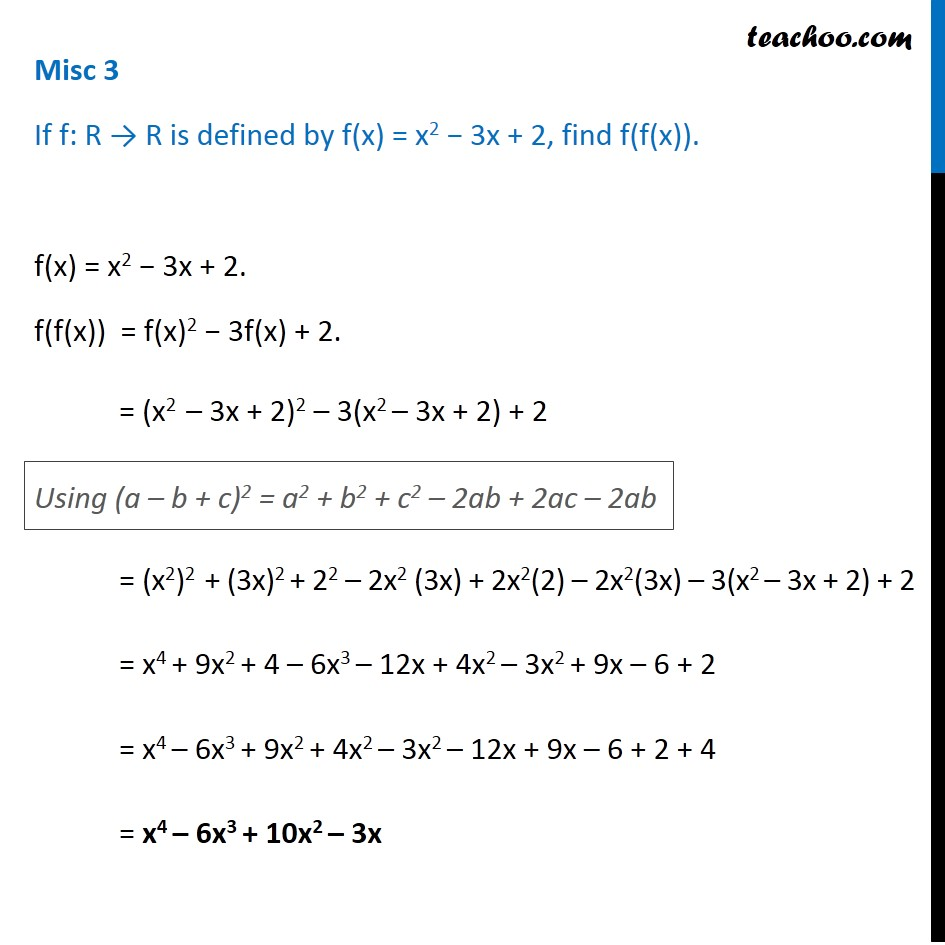Chapter 1 Class 12 Relation and Functions

Class 12
Important Questions for exams Class 12Learn in your speed, with individual attention - Teachoo Maths 1-on-1 Class

### Transcript

Question 3 If f: R → R is defined by f(x) = x2 − 3x + 2, find f(f(x)). f(x) = x2 − 3x + 2. f(f(x)) = f(x)2 − 3f(x) + 2. = (x2 – 3x + 2)2 – 3(x2 – 3x + 2) + 2 = (x2)2 + (3x)2 + 22 – 2x2 (3x) + 2x2(2) – 2x2(3x) – 3(x2 – 3x + 2) + 2 = x4 + 9x2 + 4 – 6x3 – 12x + 4x2 – 3x2 + 9x – 6 + 2 = x4 – 6x3 + 9x2 + 4x2 – 3x2 – 12x + 9x – 6 + 2 + 4 = x4 – 6x3 + 10x2 – 3x Using (a – b + c)2 = a2 + b2 + c2 – 2ab + 2ac – 2ab